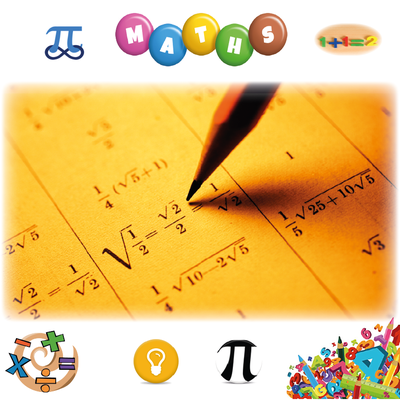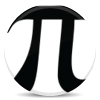## Learn ﻿﻿﻿Mathematics

An easy way to learn Mathematics online for free. Learn Maths Basics & Prealgebra; Geometry, Algebra & Trigonometry; Precalculus, Calculus & much more through this very simple course. Multiple Choice Tests. Thanks to Mem creators, Contributors & Users.
64.9k estudiantes Duración media 286h### 100 x 100  Multiplication Table

30.2k 81h### Maths GCSE

16.5k 50mMaths

### Learn Times Tables

16.2k 1h### Multiplication Tables

13.8k 2hMaths

### Learn ﻿﻿﻿﻿﻿﻿﻿Statistics

12k 23h### 'Basic Trigonometric Identities'

11.9k 35m### Probability Theory and Statistics (Introduction)

6.8k 7h### 100 Perfect Squares

6.16k 2h### A level Edexcel Maths and Further Maths

6.14k 2h### 150 Digits of Pi

5.43k 37m### Edexcel D1 Definitions

4.84k 25m### General Maths (Methods) CAS VCE - Trigonometry

3.74k 21mMaths

### 1 - 144 Times Tables! Multiplication and division

3.61k 5hMaths

### Conversions

3.24k 19m3.1k 22m